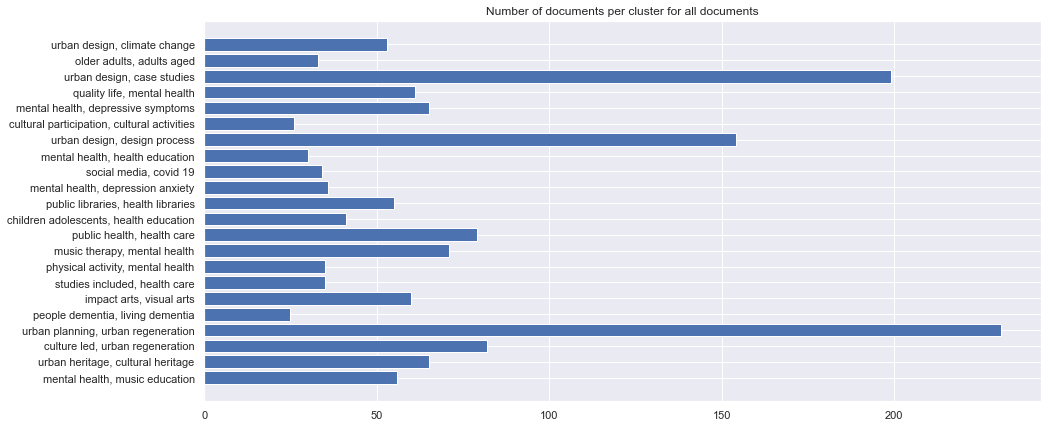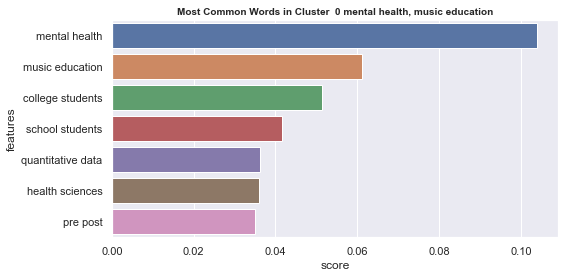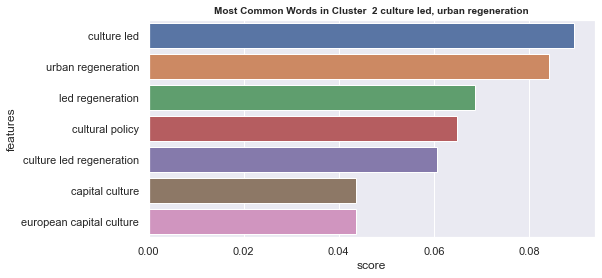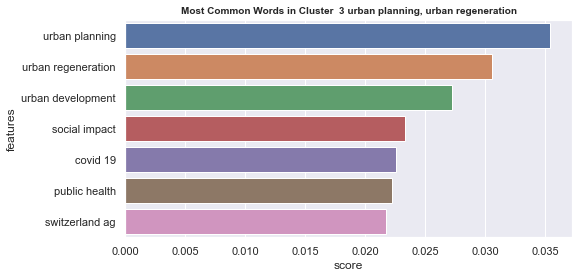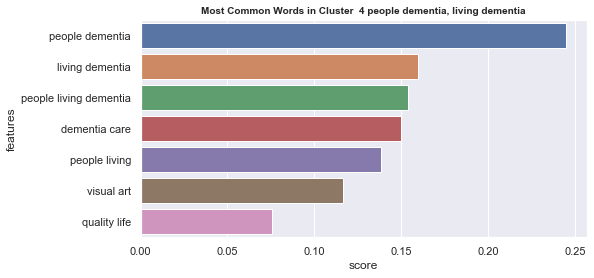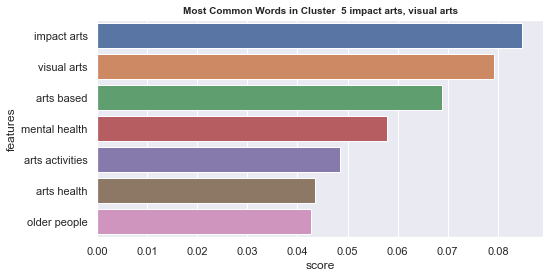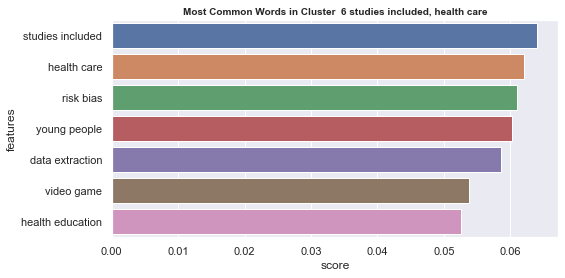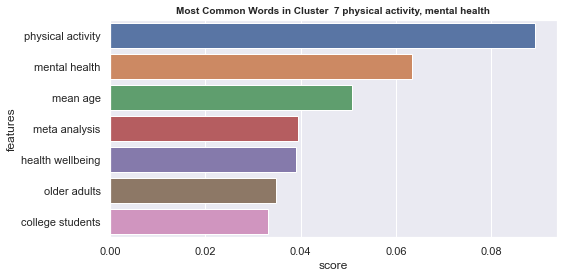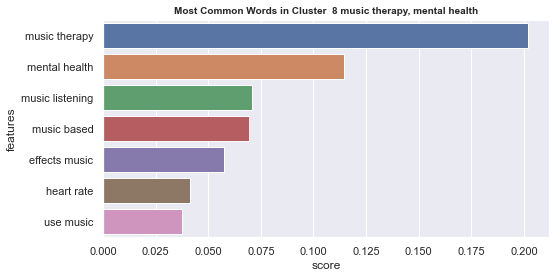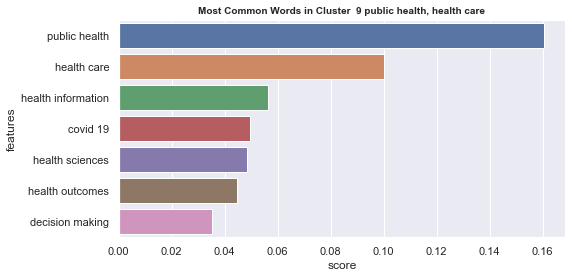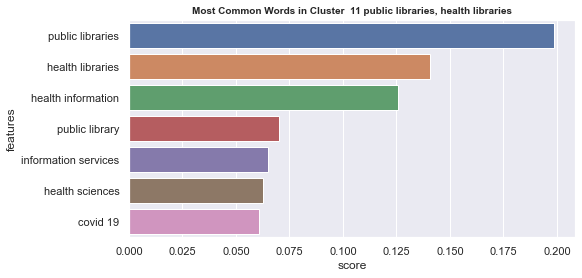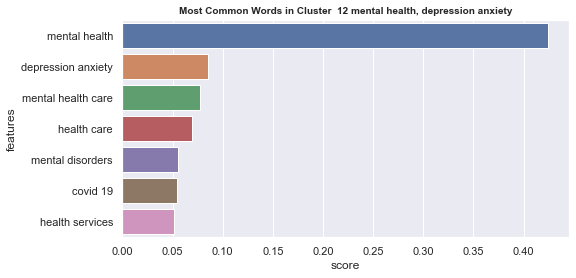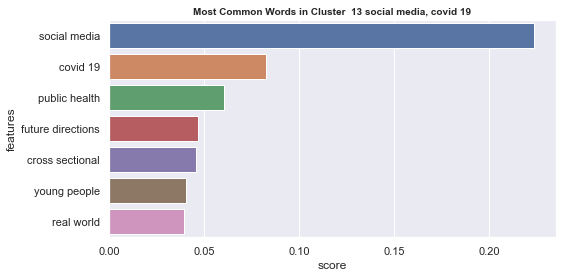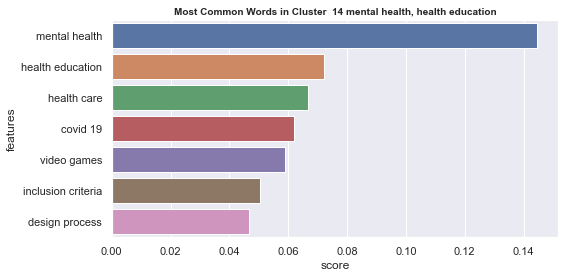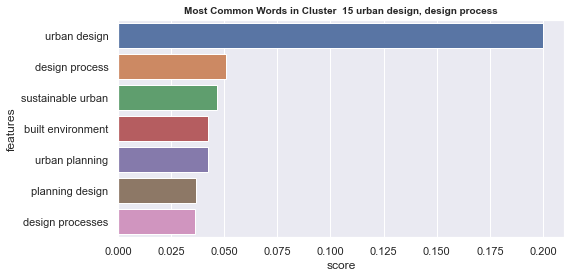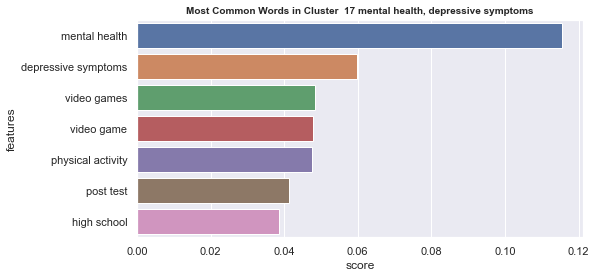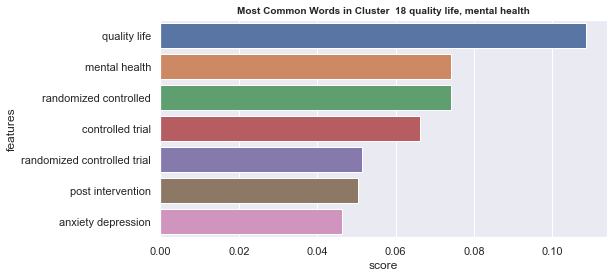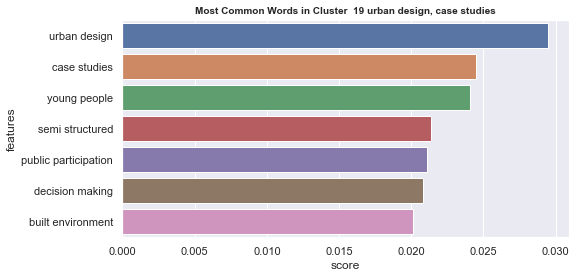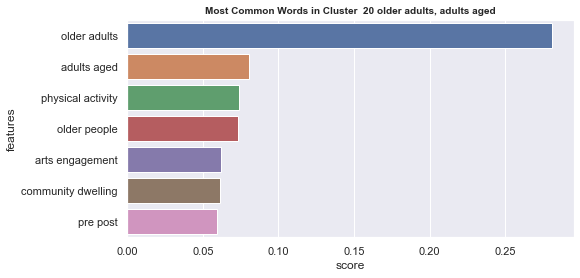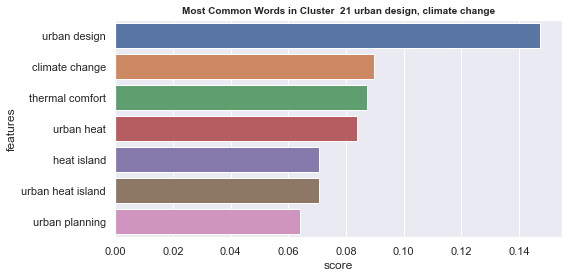# DOCUMENT CLUSTER

Cluster analysis of full text documents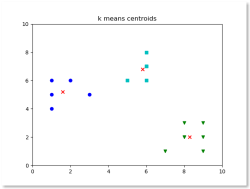### Text Clustering

Text clustering is the task of grouping a set of unlabelled texts in such a way that texts in the same cluster are more similar to each other than to those in other clusters. Text clustering algorithms process text and determine if natural clusters (groups) exist in the data.
As part of unsupervised learning, clustering is used to group similar data points without knowing which cluster the data belong to. So in a sense, document clustering is about how similar documents (or sentences) are grouped together.
Computers only calculate numbers, so we translate our texts into numbers! Essentially, what word embedding do is represent words as vectors in a space where similar words are mapped near each other. We transform each word in 4096-dimensional vector. Then K-means is used as unsupervised learning algorithm that allows us to identify similar documents. It is based on distance between text and cluster center point ( named centroid). Algorithm calculates optimal position of centroid and documents assigned to it as nearest centroid.

Initial analyisis has shown that optimum number of clusters is 22, and they are shown on following image. Each point represents one document, and clusters are represented by different colour.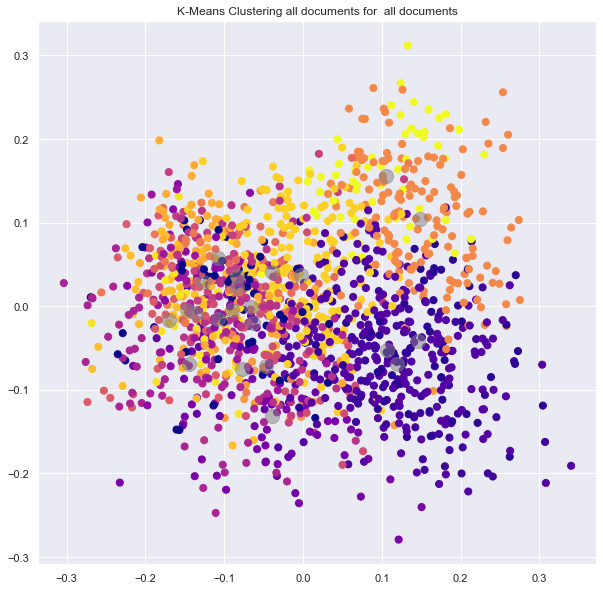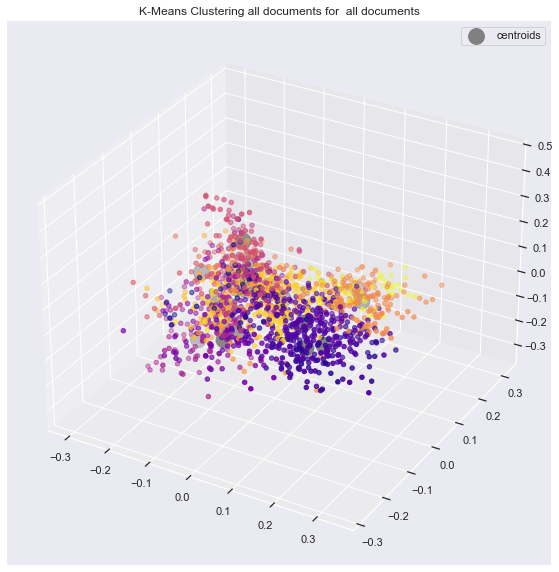This can be better viewed in 3 dimensions on following picture.
Please not that calculation has been done on 4096 dimensions.

Number of documents per cluster is visible on following picture: